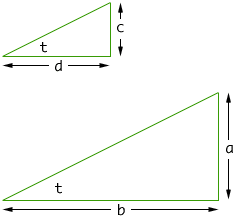Subject: Triangles Hi - If I have a right triangle, and I know the lengths of all three sides, is there a formula that will allow me to determine the measurements of the 2 non right angles? Thanks Mose Student Middle School Hi Mose, In fact if you know only two sides of a right triangle then, by Pythagoras' Theorem, you can calculate the third side. Once you know all three sides the triangle is completely determined so you should be able to deduce the size of the other two angles. This is what trigonometry can do for you. Consider the two right triangle in the diagram below, each of which has an angle measuring t o.Since the triangles are similar, a/b = c/d. This ratio is called the tangent of the angle t, and is written tan(t) = a/b. The tangent is one of the functions you learn in trigonometry. It is a function on all scientific calculators. If you input the angle measurement and push the Tan button you get the tangent of the angle. In your question, however, you know a/b and you want to find t. That is, find the angle whose tangent is a/b. This is called the inverse tangent function and can also be found with a scientific calculator. The button probably has the label tan-1 or atn. If you input the ratio a/b and push the tan-1 button the calculator will return the angle measurement. One last warning. Angles are sometimes measured in degrees and sometimes in radians. If you are using a calculator to find the tangent or the inverse tangent make sure that the calculator is set to use degrees. Cheers, Harley Go to Math Central